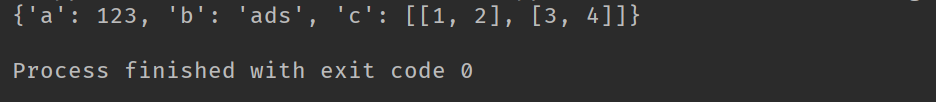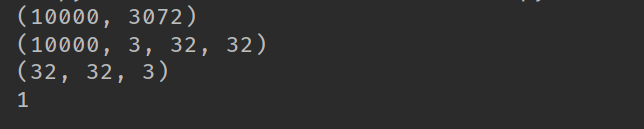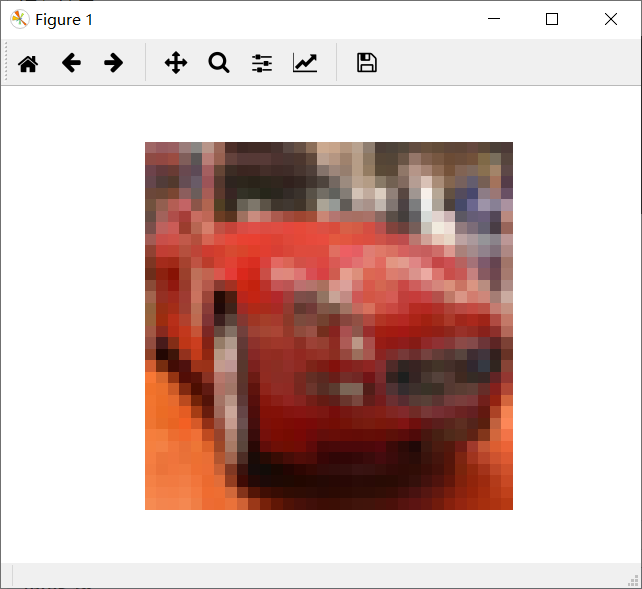# python——pickle模块的详解

pickle模块详解

pickle模块实现了用于序列化和反序列化Python对象结构的二进制协议。 “Pickling”是将Python对象层次结构转换为字节流的过程， “unpickling”是反向操作，从而将字节流（来自二进制文件类似字节的对象）转换回对象层次结构。pickle模块对于错误或恶意构造的数据是不安全的。

pickle协议和JSON（JavaScript Object Notation）的区别 ：

1. JSON是一种文本序列化格式（它输出unicode文本，虽然大部分时间它被编码utf-8），而pickle是二进制序列化格式;

2. JSON是人类可读的，而pickle则不是;

3. JSON是可互操作的，并且在Python生态系统之外广泛使用，而pickle是特定于Python的;

pickle 数据格式是特定于Python的。它的优点是没有外部标准强加的限制，例如JSON或XDR（不能代表指针共享）; 但是这意味着非Python程序可能无法重建pickled Python对象。

pickle模块提供以下常量：

pickle.HIGHEST_PROTOCOL

pickle.DEFAULT_PROTOCOL

pickle模块提供以下功能，使酸洗过程更加方便：

pickle.dump（obj，file，protocol = None，*，fix_imports = True

pickle.dumps（obj，protocol = None，*，fix_imports = True

pickle.load（file，*，fix_imports = True，encoding =“ASCII”，errors =“strict”

pickle的协议版本是自动检测的，因此不需要协议参数。超过pickle对象的表示的字节将被忽略。

pickle.loads（bytes_object，*，fix_imports = True，encoding =“ASCII”，errors =“strict”

bytes对象读取pickle对象层次结构并返回其中指定的重构对象层次结构。

pickle的协议版本是自动检测的，因此不需要协议参数。超过pickle对象的表示的字节将被忽略。

import numpy as np
import pickle
import io

if __name__ == '__main__':
path = 'test'
f = open(path, 'wb')
pickle.dump(data, f)
f.close()

f1 = open(path, 'rb')
print(data1)import numpy as np
import pickle
import random
import matplotlib.pyplot as plt
from PIL import Image

path1 = 'D:\\tmp\cifar10_data\cifar-10-batches-py\data_batch_1'
path2 = 'D:\\tmp\cifar10_data\cifar-10-batches-py\data_batch_2'
path3 = 'D:\\tmp\cifar10_data\cifar-10-batches-py\data_batch_3'
path4 = 'D:\\tmp\cifar10_data\cifar-10-batches-py\data_batch_4'
path5 = 'D:\\tmp\cifar10_data\cifar-10-batches-py\data_batch_5'

path6 = 'D:\\tmp\cifar10_data\cifar-10-batches-py\\test_batch'

if __name__ == '__main__':
with open(path1, 'rb') as fo:

# print(data[b'batch_label'])
# print(data[b'labels'])
# print(data[b'data'])
# print(data[b'filenames'])

print(data[b'data'].shape)

images_batch = np.array(data[b'data'])
images = images_batch.reshape([-1, 3, 32, 32])
print(images.shape)
imgs = images[5, :, :, :].reshape([3, 32, 32])
img = np.stack((imgs[0, :, :], imgs[1, :, :], imgs[2, :, :]), 2)

print(img.shape)

plt.imshow(img)
plt.axis('off')
plt.show()posted @ 2019-06-08 09:55  Baby-Lily  阅读(103516)  评论(2编辑  收藏  举报